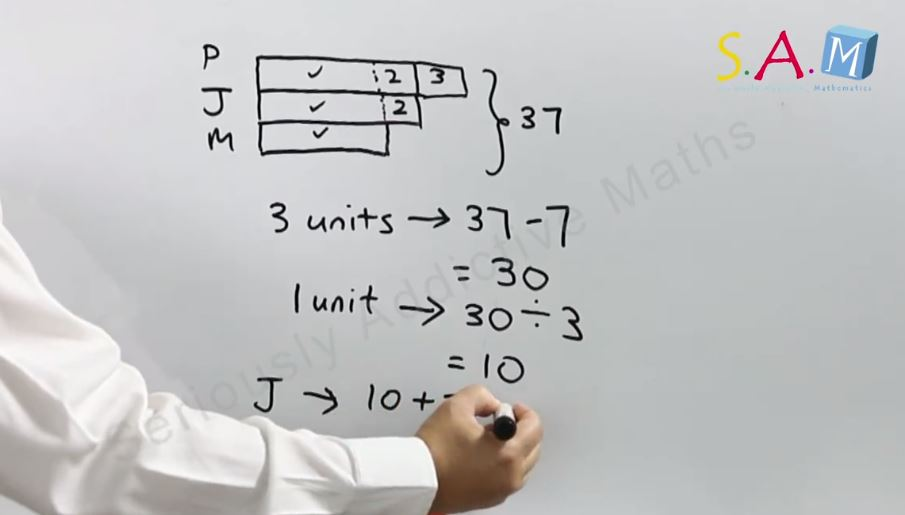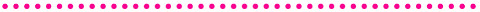Do you have trouble teaching your child the bar modelling method? Here are some easy video tutorials from Seriously Addictive Maths to help you.1. Introduction to Bar Modelling: Using it to solve arithmetic problem sums2. Fractions: How to use the bar model method to solve fractions problem sums?3. Ratio: How do you use the bar model method to solve ratio problem sums?4. Percentage: Using the bar modelling method to solve problem sums on percentage5. Basic Math Concepts: How to use the bar modeling method to solve skip counting, number line (more than), place values, multiplication, division and fractions questions